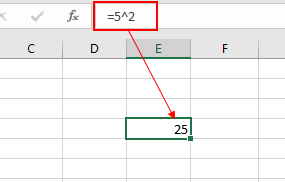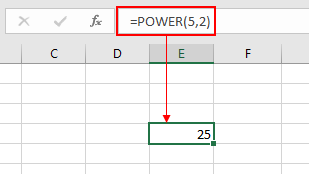# Exponents in Excel

In simple words, the exponent of a number is, the number of times the number gets multiplied.

= 23  (In Mathematics) = 8

= 2 ^ 3 (In Excel) =  8

There are 2 ways to multiply numbers in Excel.

1. Use the Caret character ( ^)
2. Excel POWER function

Let's learn about them one by one:

Caret ( ^ ) sign is used to indicate the exponent of a number. To use this method first start with equals ( = ) sign  and then use the caret ( ^ ) sign between the numbers and exponent (power).As shown in the above snapshot, the 2 Power of 5 comes out to be 25, which is 5 x 5.

The second method is using the Excel mathematical formula, The POWER function.

The POWER function calculates the power of a number.

Syntax:

=POWER ( number, power)As you can see from the above snapshot that POWER function returns the same result as the excel exponent character ( ^ ) does.

Notes:

1. The function returns the error if the argument to the function is non numeric.

Hope this article about how to Exponents in Excel is explanatory. Find more articles on SUMPRODUCT functions here. Please share your query below in the comment box. We will assist you.

Related Articles

How to use the SUMPRODUCT function in Excel

How to use the PRODUCT function in Excel

Validation of text entries

Create drop down list in excel with colour

Remove leading and trailing spaces from text in Excel

Popular Articles

50 Excel Shortcut to Increase Your Productivity

Edit a dropdown list

Absolute reference in Excel

If with conditional formatting

If with wildcards

Vlookup by date

Convert Inches To Feet and Inches in Excel 2016

Join first and last name in excel

Count cells which match either A or B

Terms and Conditions of use

The applications/code on this site are distributed as is and without warranties or liability. In no event shall the owner of the copyrights, or the authors of the applications/code be liable for any loss of profit, any problems or any damage resulting from the use or evaluation of the applications/code.